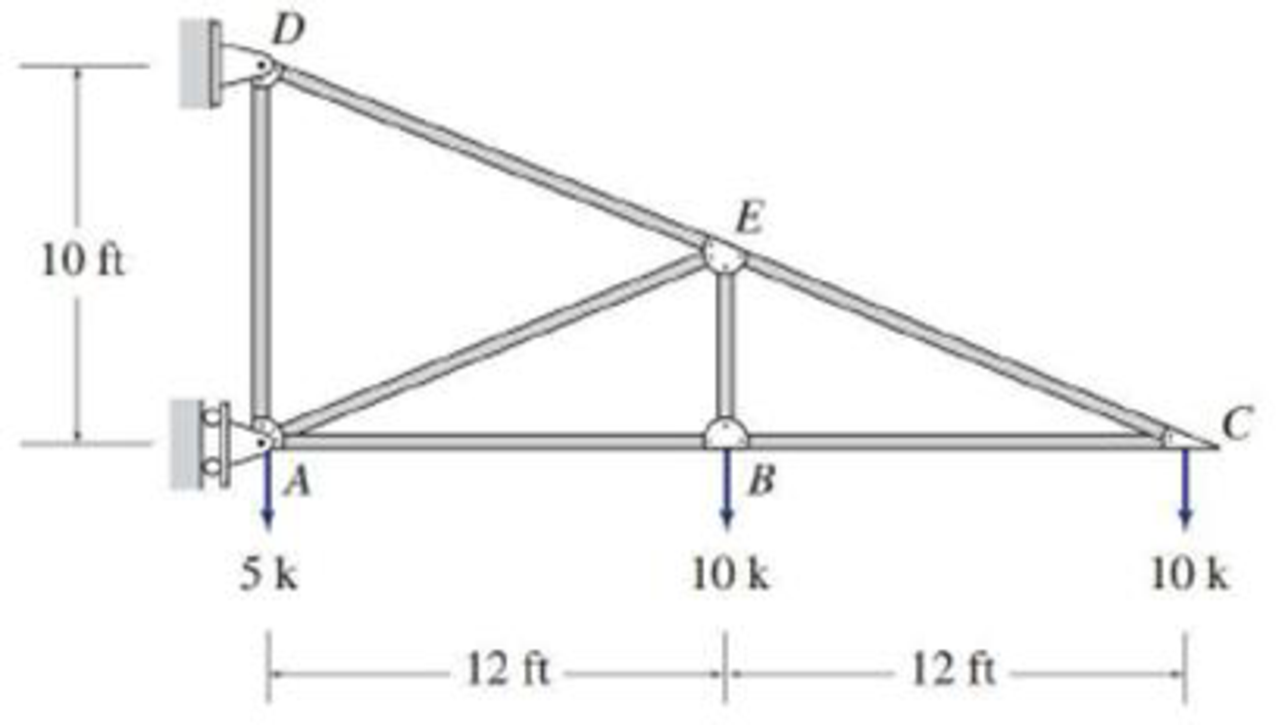# 4.6 through 4.28 Determine the force in each member of the truss shown by the method of joints. FIG P4.6

#### Solutions

Chapter
Section
Chapter 4, Problem 6P
Textbook Problem
94 views

## 4.6 through 4.28 Determine the force in each member of the truss shown by the method of joints.FIG P4.6

To determine

Find the forces in the members of the truss by the method of joints.

### Explanation of Solution

Given information:

Apply the sign conventions for calculating reactions, forces and moments using the three equations of equilibrium as shown below.

• For summation of forces along x-direction is equal to zero (Fx=0), consider the forces acting towards right side as positive (+) and the forces acting towards left side as negative ().
• For summation of forces along y-direction is equal to zero (Fy=0), consider the upward force as positive (+) and the downward force as negative ().
• For summation of moment about a point is equal to zero (Matapoint=0), consider the clockwise moment as negative and the counter clockwise moment as positive.

Method of joints:

The negative value of force in any member indicates compression (C) and the positive value of force in any member indicates Tension (T).

Consider the horizontal and vertical reactions at D are Dx and Dy.

Consider the horizontal reaction at A are Ax.

Calculation:

Show the free body diagram of the truss as shown in Figure 1.

Refer Figure 1.

For Equilibrium of forces,

Take the sum of all horizontal forces in x direction as zero.

Fx=0Dx+Ax=0Dx=Ax (1)

Take the sum of all vertical forces in y direction as zero.

Fy=0Dy51010=0Dy=25k

Take sum of moments about the support A as zero.

MA=0Dx×1010×1210×24=010Dx=360Dx=36k

Substitute 36k for Dx in Equation (1).

Ax=36k

Consider the triangle DCA.

Consider the angle DCA is θ. Then,

tanθ=1024θ=tan1(1024)θ=22.61°

Show the joint C as shown in Figure 2.

Refer Figure 2.

Take the sum of the forces acting in the vertical direction as zero. Then,

FECsinθ=10FECsin(22.61°)=10FEC=10sin(22.61°)FEC=26k(T)

Take the sum of the forces acting in the horizontal direction as zero. Then,

FBCFECcosθ=0FBC=FECcos(22.61°)FBC=26cos(22.61°)FBC=24kFBC=24k(C)

Show the joint B as shown in Figure 3.

Refer Figure 3.

Take the sum of the forces acting in the vertical direction as zero. Then,

FBE=10k(T)

Take the sum of the forces acting in the horizontal direction as zero

### Still sussing out bartleby?

Check out a sample textbook solution.

See a sample solution

#### The Solution to Your Study Problems

Bartleby provides explanations to thousands of textbook problems written by our experts, many with advanced degrees!

Get Started

Find more solutions based on key concepts
Collect information on the American Wire Gage (AWG) standards. Create a table for annealed copper wires showing...

Engineering Fundamentals: An Introduction to Engineering (MindTap Course List)

An aspect of cloud computing that allows developers to create, test, and run their solutions on a cloud platfor...

Enhanced Discovering Computers 2017 (Shelly Cashman Series) (MindTap Course List)

What is an InfoSec framework?

Management Of Information Security

What is the purpose of the master cylinder vent port?

Automotive Technology: A Systems Approach (MindTap Course List)

Resolve the 360-lb force into components along the cables AB and AC. Use =60 and =40.

International Edition---engineering Mechanics: Statics, 4th Edition

What are optimizer hints, and how are they used?

Database Systems: Design, Implementation, & Management

Define an attribute, and provide three examples.

Systems Analysis and Design (Shelly Cashman Series) (MindTap Course List)

Convert the ERD from Problem 10 into a corresponding UML class diagram.

Database Systems: Design, Implementation, & Management

Where should you stand when using a grinder?

Precision Machining Technology (MindTap Course List)

If your motherboard supports ECC DDR3 memory, can you substitute non-ECC DDR3 memory?

A+ Guide to Hardware (Standalone Book) (MindTap Course List)

What changes can be made to successfully make a weld in a poorly fitted joint?

Welding: Principles and Applications (MindTap Course List)